# For - Next Statement in QBASIC

## State whether the following statements are True/False

#### Question 1

FOR statement may or may not be followed by NEXT.
False

#### Question 2

FOR-NEXT statement is used to generate finite loops.
True

#### Question 3

A loop can also be generated using the GOTO statement.
False

#### Question 4

The initial value of the variable FOR may begin with a negative number.
True

#### Question 5

By default, the increment of the variable in a FOR-NEXT statement is +1.
True

#### Question 6

Without using the STEP value, the control may jump to the next value.
True

#### Question 1

What is a loop?

A loop is a conditional repetitive type of flow and the program runs until the condition is satisfied. A task repeated again and again for a fixed number of times is known as a loop.

#### Question 2

What are the different ways to generate a loop in BASIC programming?

In BASIC programming, we can generate a loop by using:

1. Counter method
2. WHILE-WEND loop
3. FOR-NEXT loop
4. Do WHILE loop

#### Question 3

Explain the following with an example:
(a) Continuous loop
(b) Step loop

(a) Continuous loop: If the increment of the loop variable is by one, then it is known as a continuous loop.

Example:

``````CLS
FOR I = 1 TO 10
PRINT "CONTINUOUS LOOP"
NEXT I
END
``````

(b) Step loop: If the increment of the loop variable is defined by STEP and the value is other than one, then it is known as a step loop.

Example:

``````CLS
FOR I = 1 TO 10 STEP 2
PRINT "CONTINUOUS LOOP"
NEXT I
END
``````

#### Question 4

Write down the format of:
(a) WHILE - WEND
(b) FOR-NEXT

(a) WHILE - WEND The format of WHILE - WEND is as follows:

``````WHILE <condition>
...
...
WEND
``````

(b) FOR - NEXT The format of FOR - NEXT is as follows:

``````FOR <variable> = <initial value> TO <Final value>
...
...
NEXT <variable>
``````

## QBASIC Programming using FOR-NEXT

#### Question 1a

Draw a flowchart and write a program in QBASIC to display the first 10 terms of the series 3, 6, 12, 24, .......

Flowchart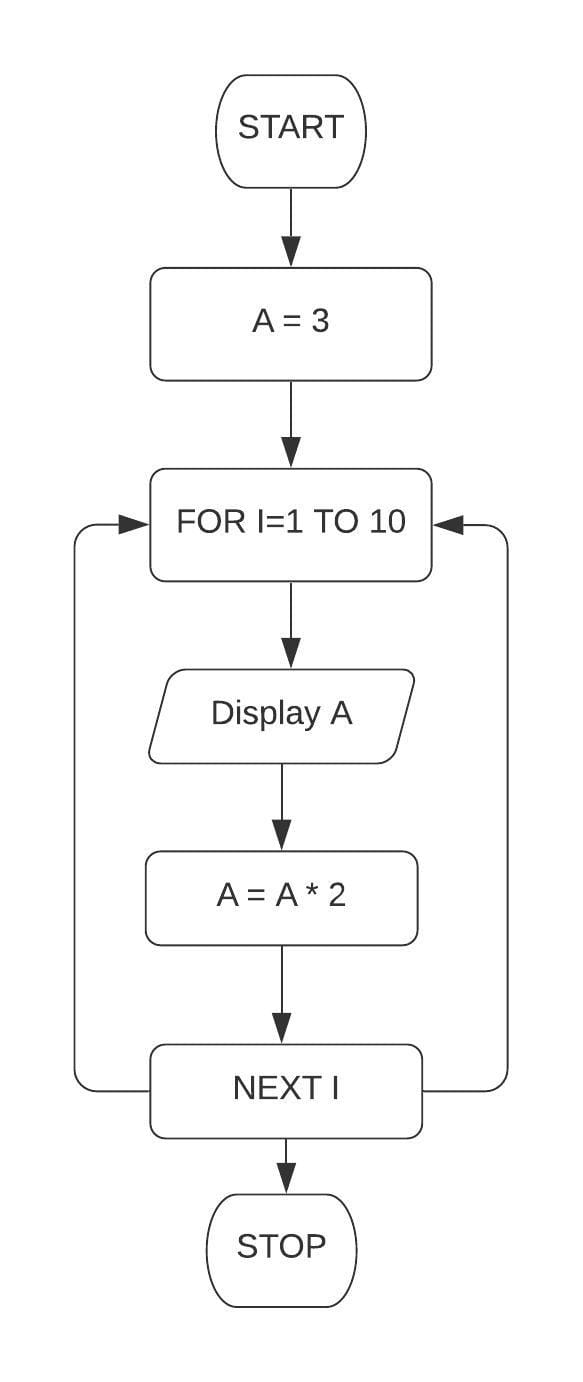QBASIC Program

``````Cls
Let A = 3
For I = 1 To 10
Print A;
A = A * 2
Next I
End
``````

Output

``````3  6  12  24  48  96  192  384  768  1536
``````

#### Question 1b

Draw a flowchart and write a program in QBASIC to display the first 10 terms of the series -1, 2, 5, 8, .......

Flowchart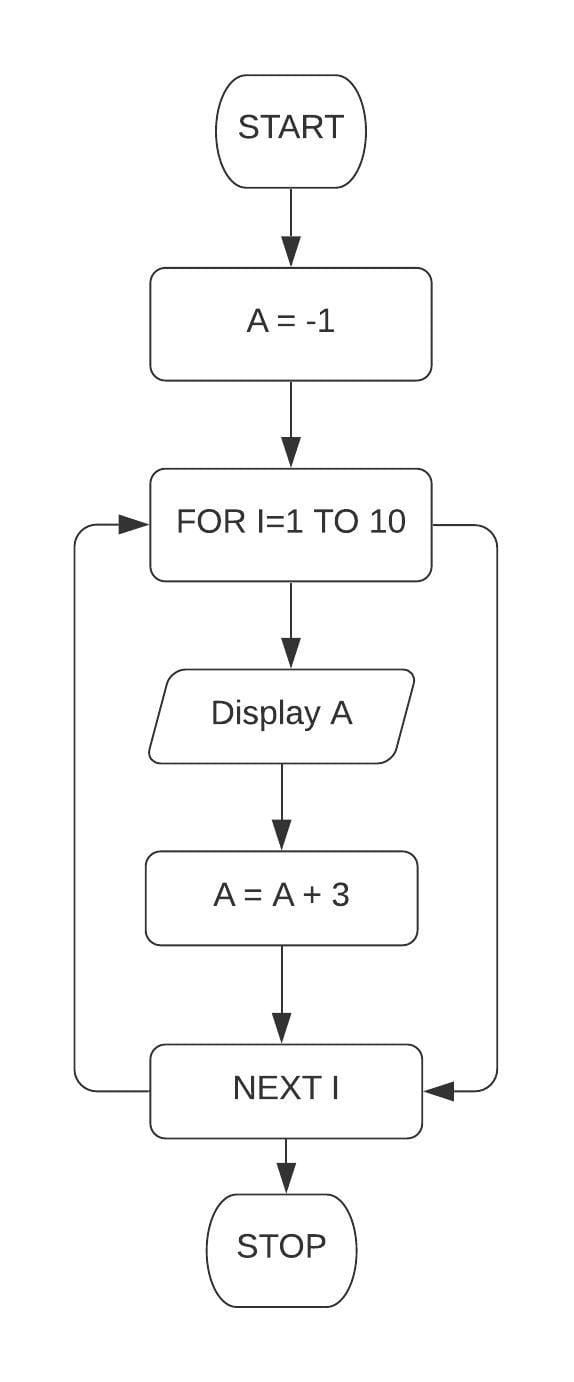QBASIC Program

``````Cls
Let A = -1
For I = 1 To 10
Print A;
A = A + 3
Next I
END
``````

Output

``````-1  2  5  8  11  14  17  20  23  26
``````

#### Question 1c

Draw a flowchart and write a program in QBASIC to display the first 10 terms of the series 7, 14, 21, .......

Flowchart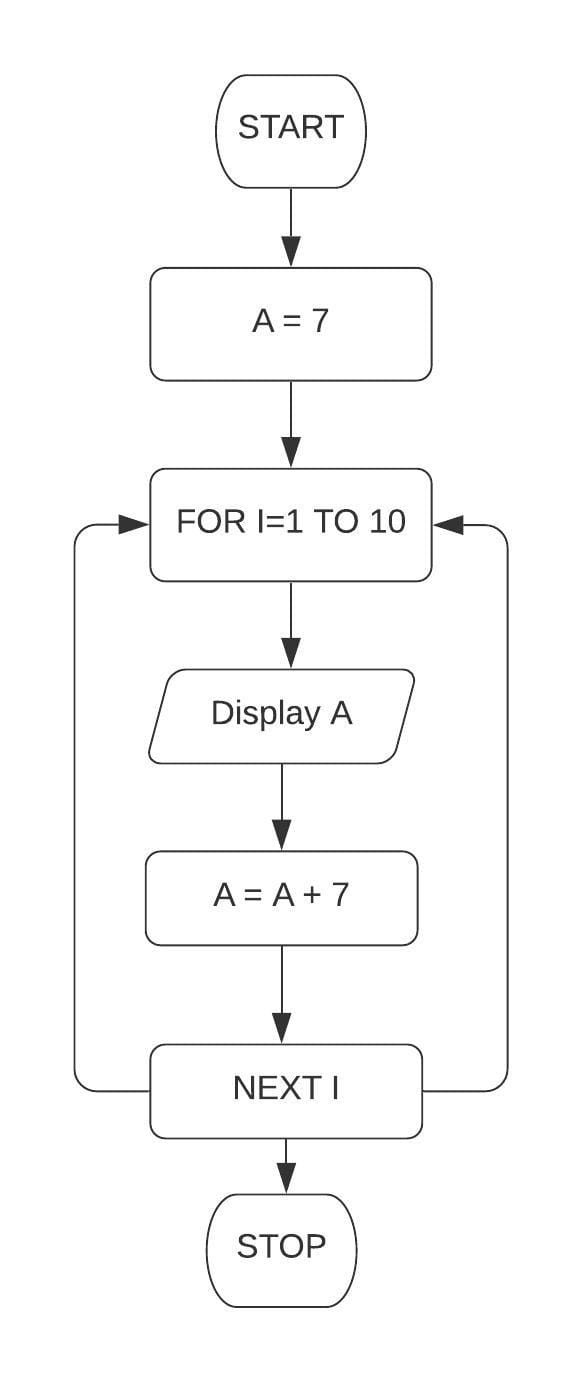QBASIC Program

``````Cls
Let A = 7
For I = 1 To 10
Print A;
A = A + 7
Next I
End
``````

Output

``````7  14  21  28  35  42  49  56  63  70
``````

#### Question 2a

Draw a flowchart and write a program in QBASIC to display the sum of the first 10 terms of the series S = 5 + 8 + 11 + .......

Flowchart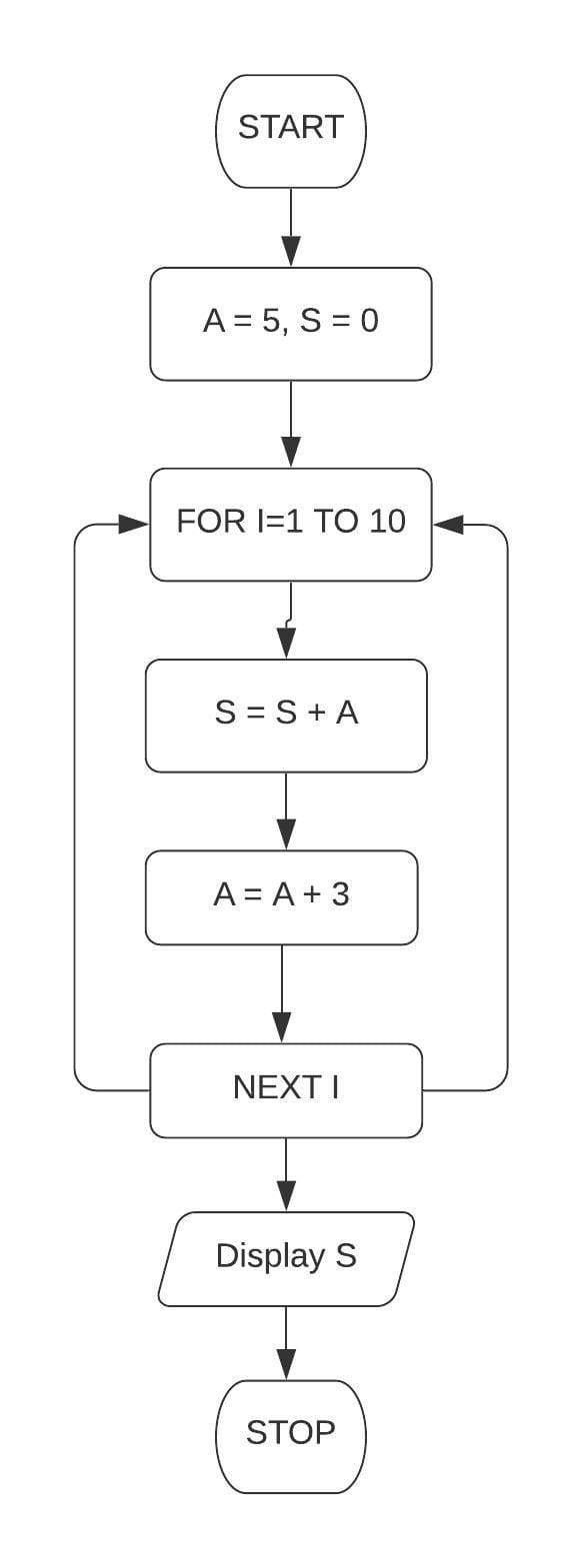QBASIC Program

``````Cls
Let A = 5
Let S = 0
For I = 1 To 10
S = S + A
A = A + 3
Next I
Print "Sum = "; S
End
``````

Output

``````Sum = 185
``````

#### Question 2b

Draw a flowchart and write a program in QBASIC to display the sum of the first 10 terms of the series S = 99 + 90 + 81 + .......

Flowchart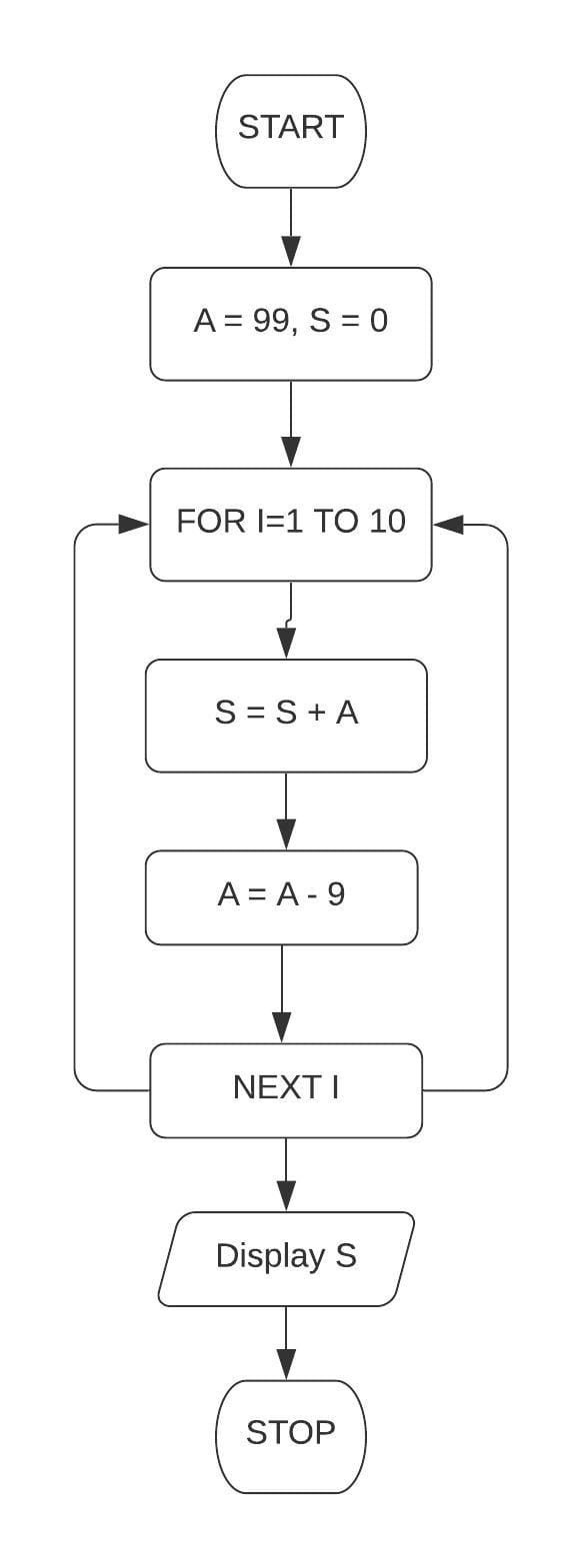QBASIC Program

``````Cls
Let A = 99
Let S = 0
For I = 1 To 10
S = S + A
A = A - 9
Next I
Print "Sum = "; S
End
``````

Output

``````Sum = 585
``````

#### Question 2c

Draw a flowchart and write a program in QBASIC to display the sum of the first 10 terms of the series S = 1 + 2 + 4 + 8 + .......

Flowchart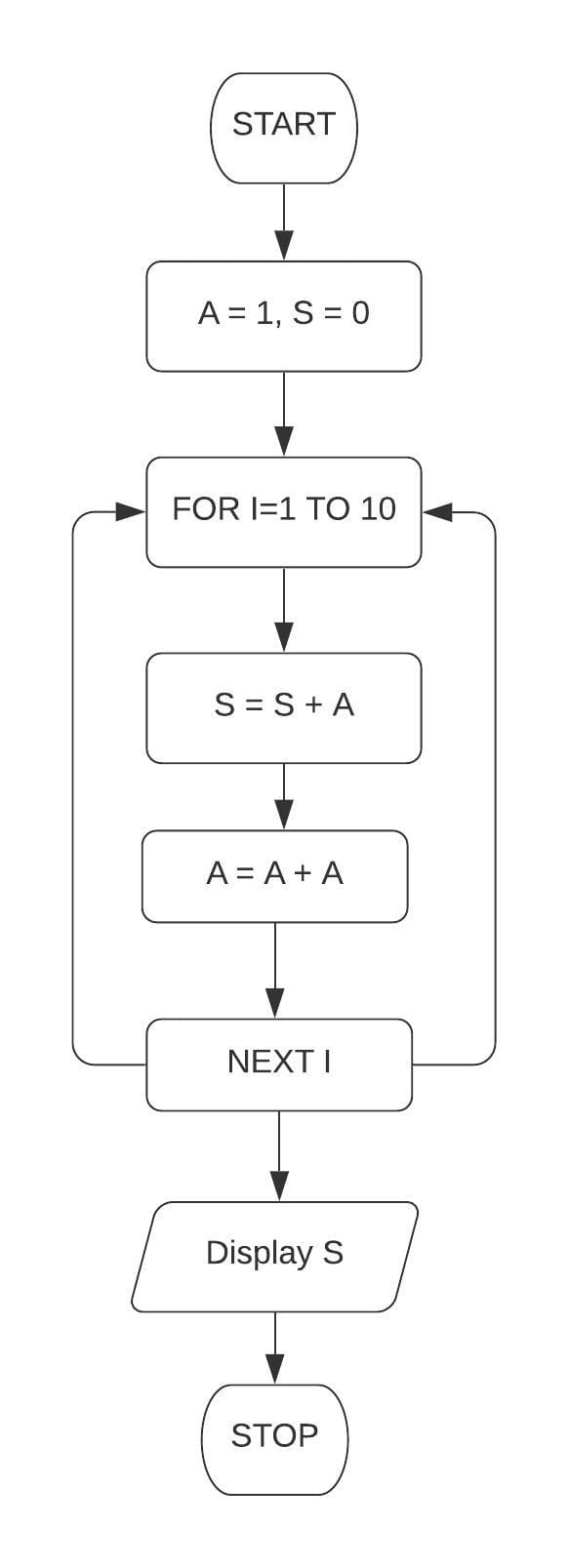QBASIC Program

``````Cls
Let A = 1
Let S = 0
For I = 1 To 10
S = S + A
A = A + A
Next I
Print "Sum = "; S
End
``````

Output

``````Sum = 1023
``````

#### Question 3

Draw a flowchart and write a program in QBASIC to enter the name and marks secured in 12 subjects in a term examination. Display the name, total and the average marks.

Flowchart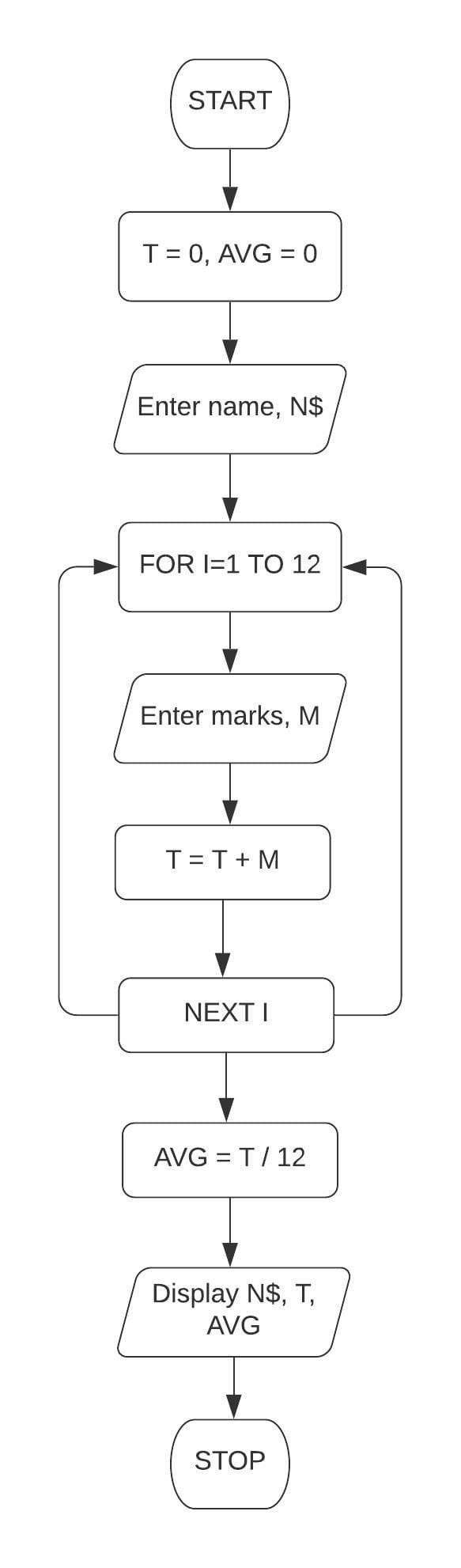QBASIC Program

``````Cls
Let T = 0
Let AVG = 0
Input "Enter name"; N\$
For I = 1 To 12
Input "Enter marks"; M
T = T + M
Next I
AVG = T / 12
Print "Name - "; N\$
Print "Total marks -"; T
Print "Average marks - "; AVG
End
``````

Output

``````Enter name? Richa
Enter marks? 89
Enter marks? 89
Enter marks? 87
Enter marks? 90
Enter marks? 99
Enter marks? 95
Enter marks? 97
Enter marks? 92
Enter marks? 78
Enter marks? 95
Enter marks? 78
Enter marks? 80
Name - Richa
Total marks - 1069
Average marks - 89.08334
``````

#### Question 4

Draw a flowchart and write a program in QBASIC to calculate the area and the perimeter of 10 different rectangles. Take the length and breadth as inputs and display the result.

FlowchartQBASIC Program

``````Cls
For I = 1 To 10
Input "Enter length and breadth"; L, B
A = L * B
P = 2 * (L + B)
Print "Area = "; A
Print "Perimeter = "; P
Next I
End
``````

Output

``````Enter length and breadth? 2,1
Area = 2
Perimeter = 6
Area = 6
Perimeter = 10
Area = 8
Perimeter = 12
Area = 12
Perimeter = 14
Area = 15
Perimeter = 16
Area = 18
Perimeter = 18
Area = 20
Perimeter = 18
Area = 40
Perimeter = 26
Area = 54
Perimeter = 30
Area = 56
Perimeter = 30
``````

#### Question 5

A class teacher wants to calculate the average marks obtained by a student in three terms in a class of 50 students. Draw a flowchart and write a program in QBASIC to enter the name and the total marks obtained by a student in three terms. Display the result along with the name.

Flowchart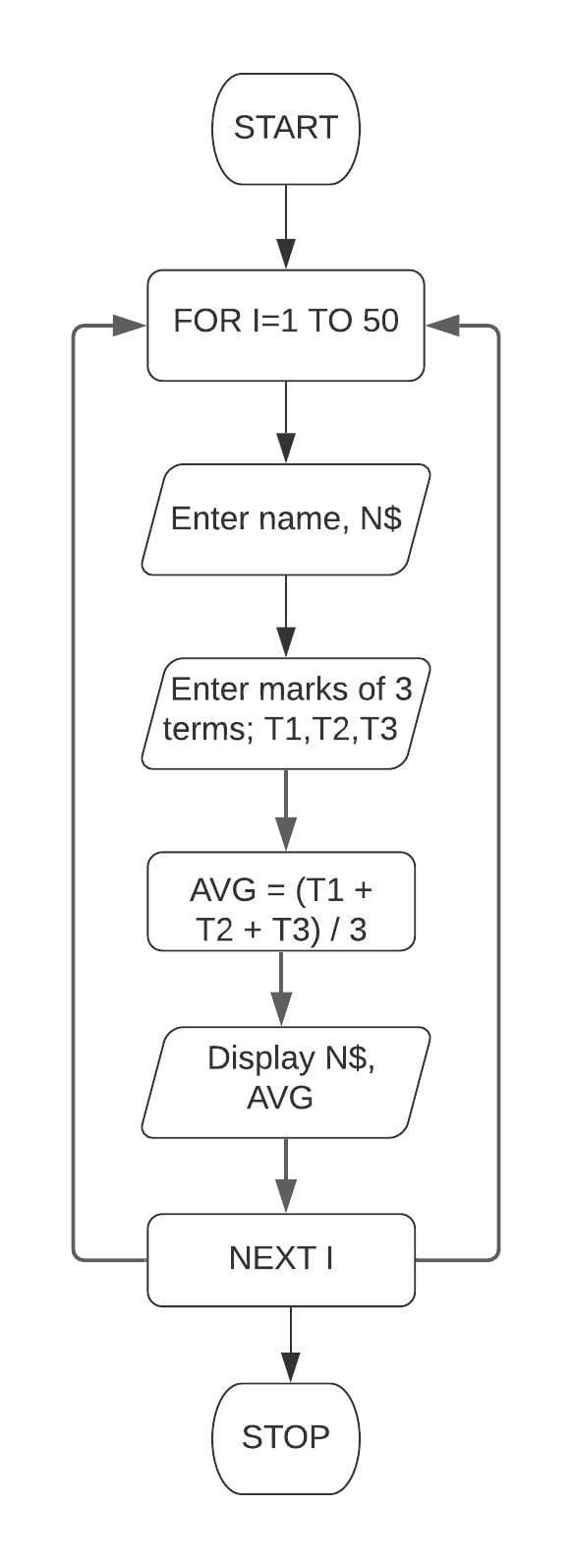QBASIC Program

``````Cls
For I = 1 To 50
Input "Enter name"; N\$
Input "Marks of three terms"; T1, T2, T3
AVG = (T1 + T2 + T3) / 3
Print "Name - "; N\$
Print "Average marks - "; AVG
Next I
End
``````

#### Question 6

Draw a flowchart and write a program in QBASIC to display 10 different values of the expression (a + b)2. Take the values of a and b as inputs after each iteration.

Flowchart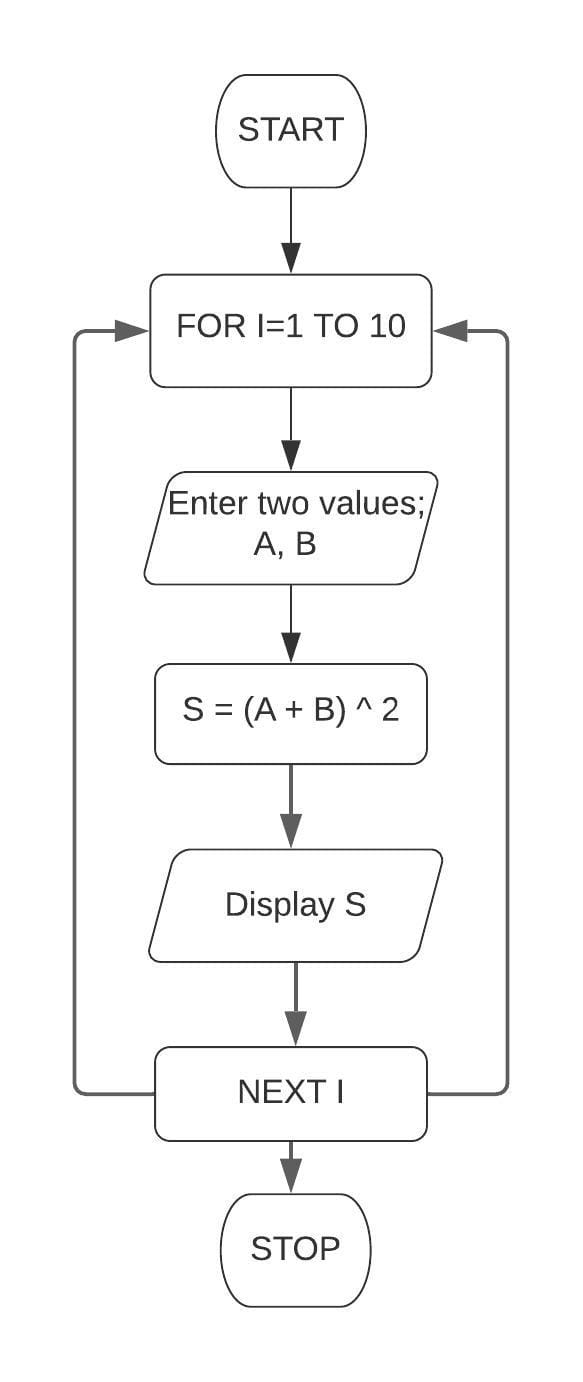QBASIC Program

``````Cls
For I = 1 To 10
Input "Enter two values"; A, B
S = (A + B) ^ 2
Print "(A+B)^2 = "; S
Next I
End
``````

Output

``````Enter two values? 2,3
(A+B)^2 = 25
Enter two values? 4,2
(A+B)^2 = 36
Enter two values? 6,3
(A+B)^2 = 81
Enter two values? 5,8
(A+B)^2 = 169
Enter two values? 3,3
(A+B)^2 = 36
Enter two values? 4,4
(A+B)^2 = 64
Enter two values? 5,5
(A+B)^2 = 100
Enter two values? 6,1
(A+B)^2 = 49
Enter two values? 4,8
(A+B)^2 = 144
Enter two values? 3,9
(A+B)^2 = 144
``````

## Correct the errors in the following flowcharts and write the programs

#### Question 1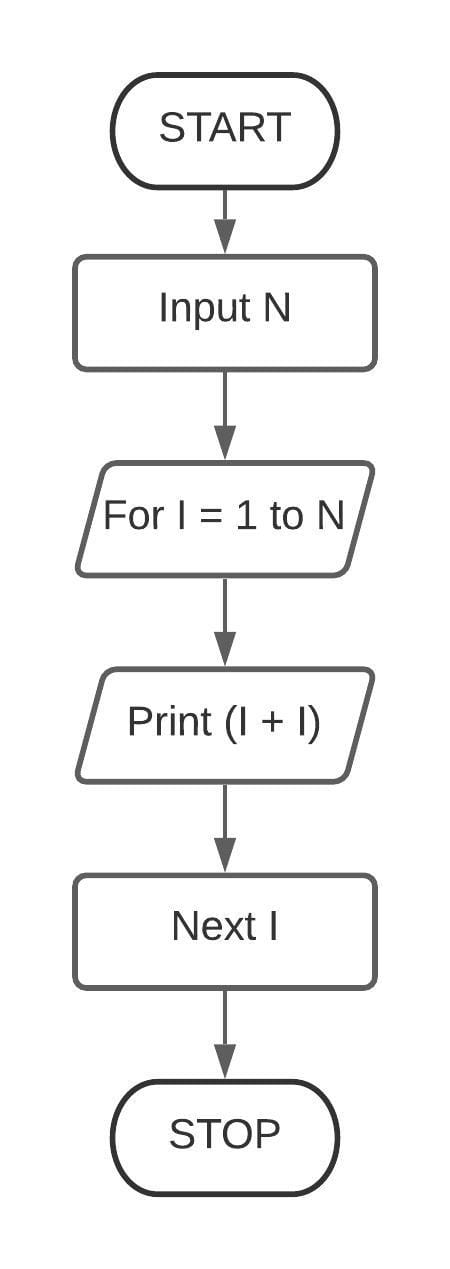Flowchart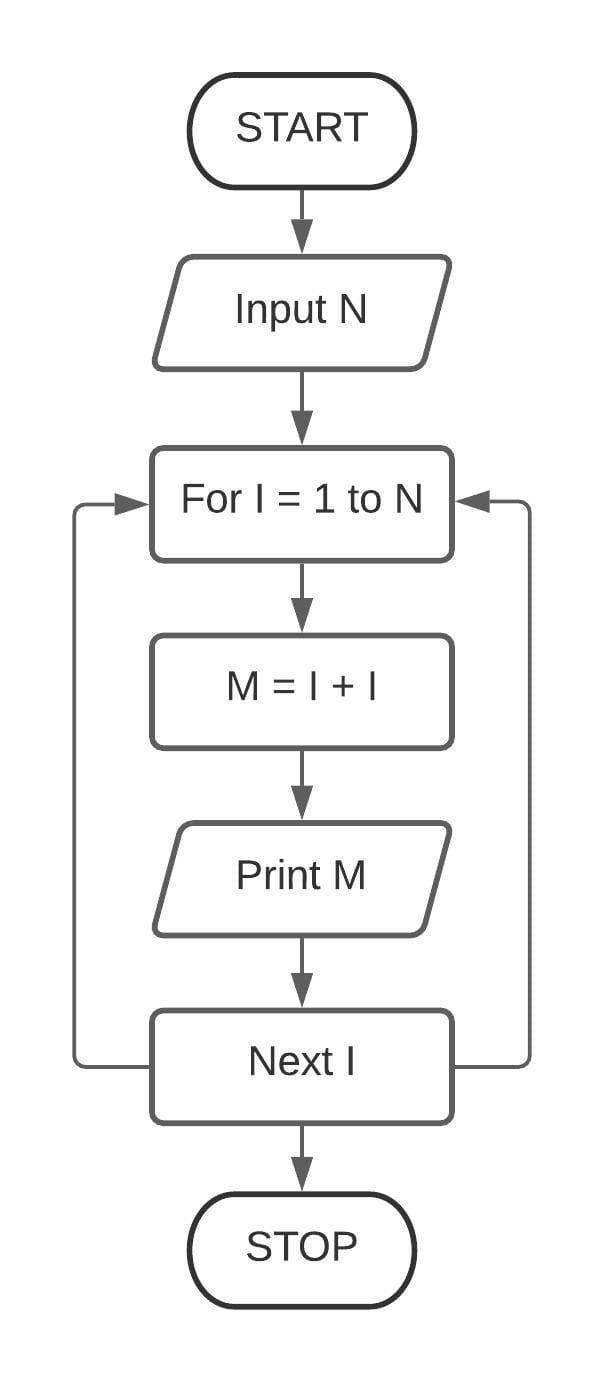QBASIC Program

``````Cls
Input "Enter a number"; N
For I = 1 To N
M = I + I
Print M
Next I
End
``````

Output

``````Enter a number? 5
2
4
6
8
10
``````

#### Question 2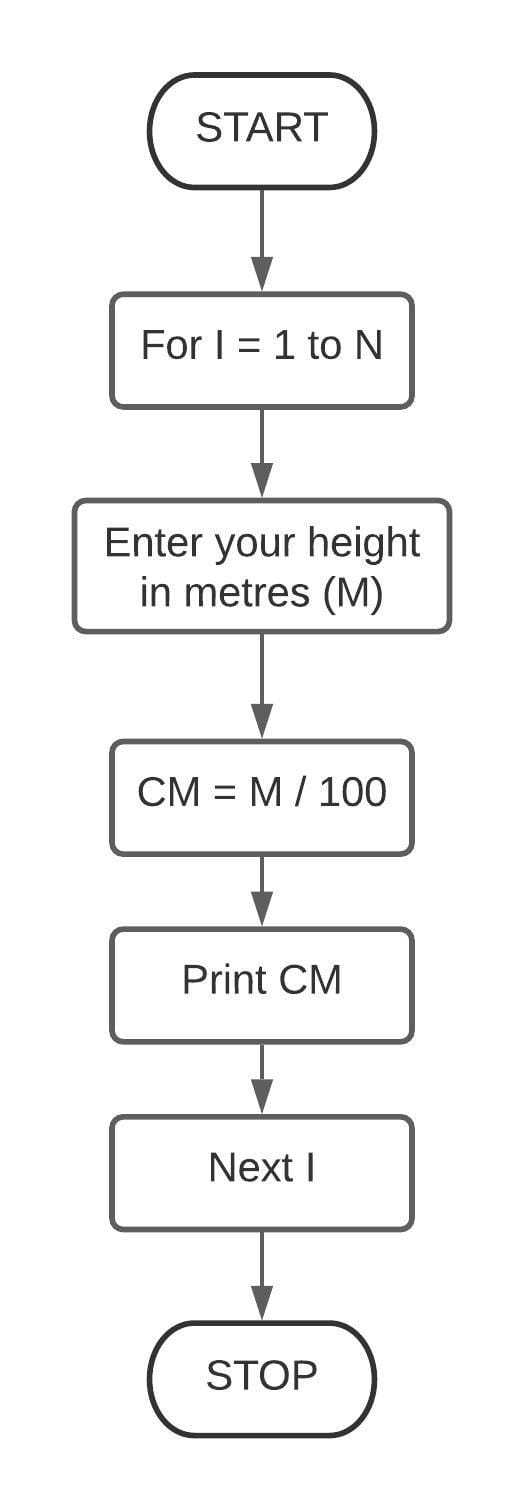Flowchart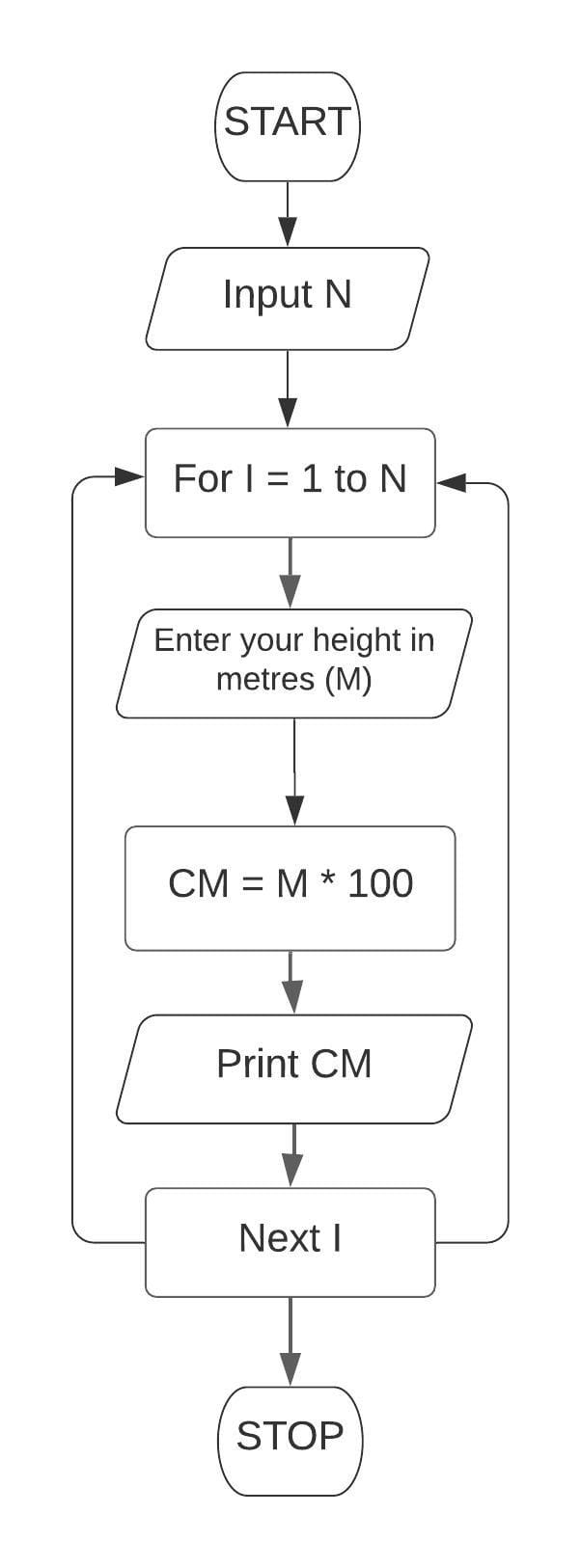QBASIC Program

``````Cls
Input "Enter a value"; N
For I = 1 To N
Input "ENTER YOUR HEIGHT IN METRES"; M
CM = M * 100
Print CM
Next I
End
``````

Output

``````Enter a value? 2
Enter your height in metres? 4
400
Enter your height in metres? 5
500
``````